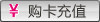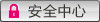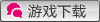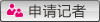活动副本 职业专栏 游戏攻略 新手百问 常用工具 游戏资料 游戏下载 独家专题 技能资料 天赋资料
当前位置： 17173网站> 鹿鼎记专区> 玩家交流> 各级护心镜原料数量及成本费

# 各级护心镜原料数量及成本费

作者： 6161616161　　此文获金币： 　　时间：2011-01-27 　　投稿得金币 　金币兑换点卡　　我要评论

【加入１７１７３鹿鼎记战地记者团得元宝】

### 文章摘要

20级护心镜=10级镜子*2

30级护心镜=20级镜子*2=10级镜子*4

40级护心镜=30级镜子*2=20级镜子*4=10级镜子*8

50级护心镜=40级镜子*2=30级镜子*4=20级镜子*8=10级镜子*16

60级护心镜=50级镜子*2=40级镜子*4=30级镜子*8=20级镜子*16=10级镜子*32

70级护心镜=60级镜子*2=50级护心镜*4=40级镜子*8=30级镜子*16=20级镜子*32=10级镜子*64

80级护心镜=70级镜子*2=60级护心镜*4=50级护心镜*8=40级镜子*16=30级镜子*32=20级镜子*64=10级镜子*128

90级护心镜=80级镜子*2=70级护心镜*4=60级护心镜*8=50级护心镜*16=40级镜子*32=30级镜子*64=20级镜子*128=10级镜子*256

100级护心镜=90级护心镜*2=80级镜子*4=70级护心镜*8=60级护心镜*16=50级护心镜*32=40级镜子*64=30级镜子*128=20级镜子*256=10级镜子*512

各等级镜子合成手工费：

10级合成20级 ： 90银

20级合成30级 ： 3金80银

30级合成40级 ： 9金50银

40级合成50级 ： 18金80银

50级合成60级 ： 28金50银

60级合成70级 ： 60金

各等级镜子合成成本：(X表示收购10级镜子价钱)

10级镜子 ： X

合成20级镜子 ： 2X+90银

合成30级镜子 ： 4X+5金60银

合成40级镜子 ： 8X+20金70银

合成50级镜子 ： 16X+60金20银

合成60级镜子 ： 32X+148金90银

合成70级镜子 ： 64X+357金80银

PS：本人财力和等级各种不足，还未收集到70级之后镜子的手续费。。还望有资本的同学不吝提供，先谢谢啦!

此贴的本意是让大家更直观的认识到合成自己所需护心镜所需的基础镜的数量和花费，至于什么“公式算多方便”“此贴真多余”之类的。在下就不再多解释啥了。如果此贴能给某位朋友帮上了那么一点点小忙，也算咱没做无用功啦。

最后，感谢 鹿呀嘛鹿鼎记 同学提供友情支援~

## 鹿鼎记热门关键字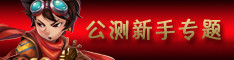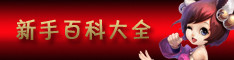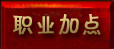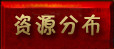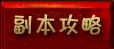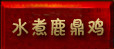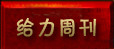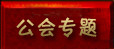## 鹿鼎记记者专访#### 专访：朱雀岛#### 专访：玄武潭

50级组金科状元，倾听状元郎圆梦经历

## 鹿鼎记官方信息